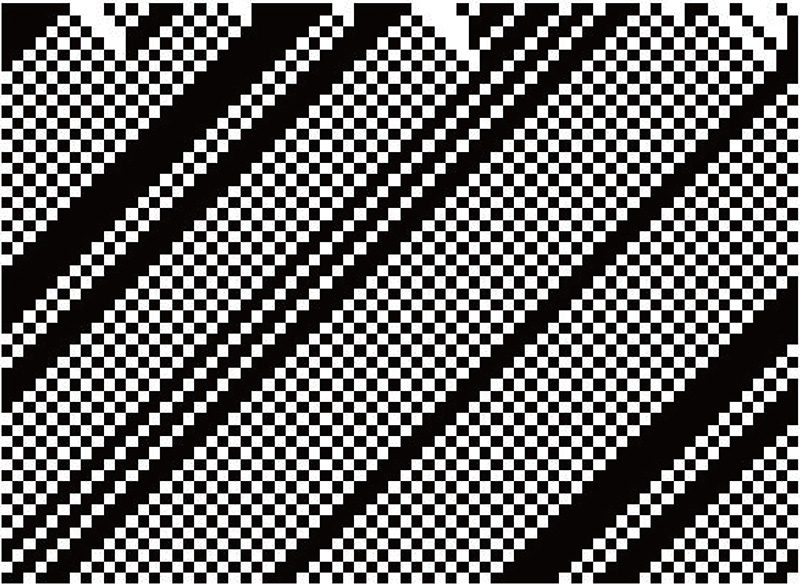# Computational Mathematics Laboratory

## Expressing complicated phenomena with simple rules

In recent years, we have seen a rapid advancement in “ultradiscretization,” a technology to identify the essential structures of natural phenomena, which are discretionally expressed in model equations. Cellular automaton — which is one of ultradiscrete equations and a completely discrete model consisting of “0s” and “1s” — is used to describe complicated phenomena such as traffic jams and ecosystems. Our laboratory conducts research on numerical calculation methods, which serve as the foundation for building mathematical models using discrete and ultradiscrete equations, in addition to numerical simulations.Affiliation Mathematical Sciences Faculty Name FUKUDA, Akiko Academic Society The Japan Society for Industrial and Applied MathematicsThe Mathematical Society of JapanInternational Linear Algebra Society Keyword Mathematical sciences, Simulations, Cellular automaton, Integrable, Information searches, Computer simulations, Differential equations, Mathematical biology, Numerical linear algebra

### Study Fields

• Mathematics
• Information science
• Applied mathematics

### For Society

Numerical simulations are indispensable when analyzing various natural and social phenomena. We will be able to reach more accurate results of analyses by developing and improving methods of numerical calculation, which serve as the foundation for numerical simulations.

### Research Themes

• Development of algorithms for calculating the eigenvalue of matrices based on the theory of discrete integrable systems
• Research on algorithms for calculating eigenvalues in max-plus algebra and their applications in network optimizations
• Research on the inverse discretization of elementary cellular automaton
• Research on speeding up the calculation of Google PageRank